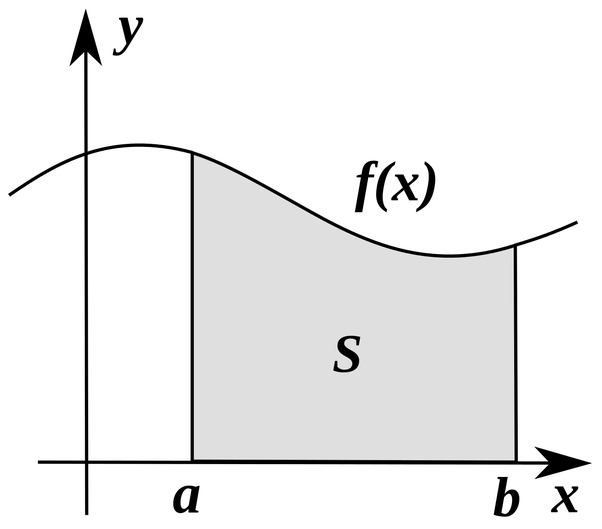# Calculation of definite integral

Numerical integration - calculation of a definite (Riemann) integral
Enter the <a, b> interval (start, stop limits) and the integrated function.

dx
The area under a curve f(x) between two points can be found by doing a definite integral between the two points. To find the area under the curve y = f(x) between x = a and x = b, integrate y = f(x) between the limits of a and b. Areas under the x-axis will come out negative and areas above the x-axis will be positive. Integral is an infinite sum of area of rectangles of infinitesimal width.⌠a
|  f(x) dx
⌡b